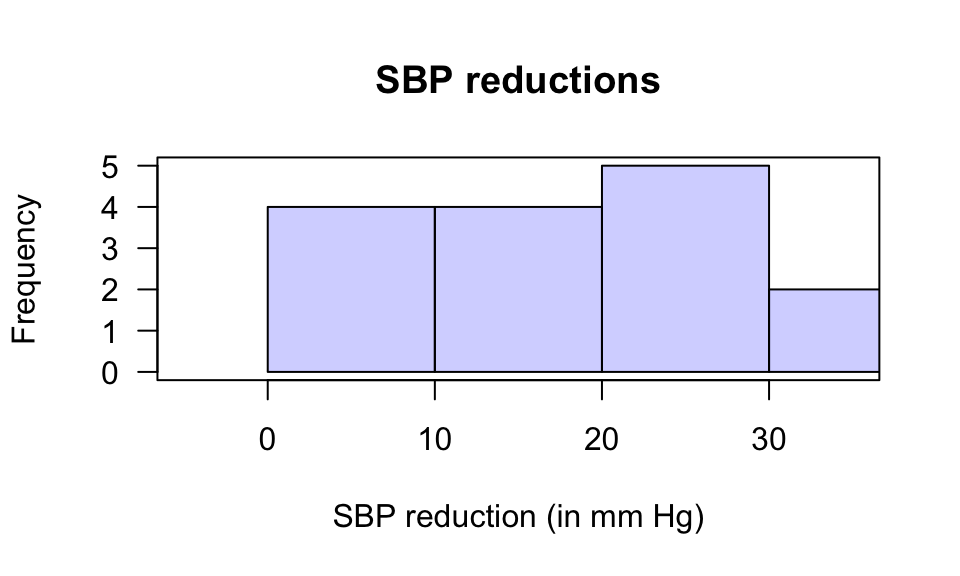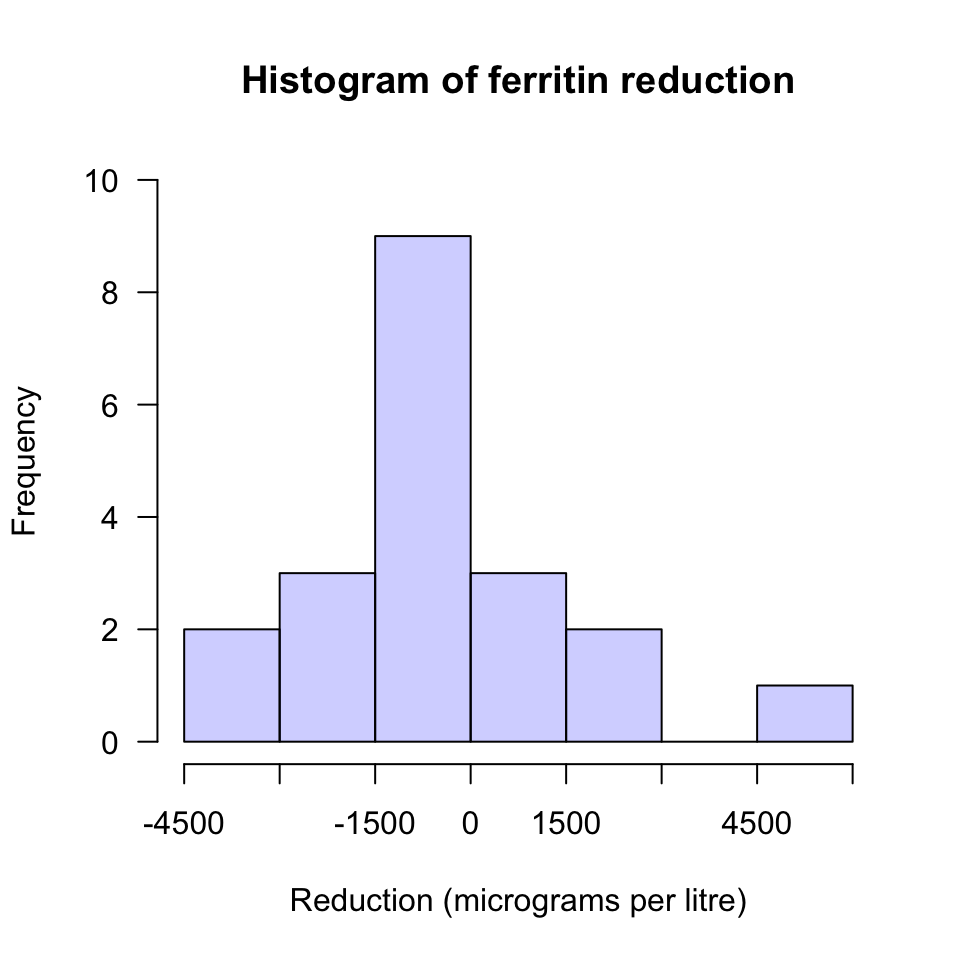## D.27 Answers: Tests for paired means

Answers to exercises in Sect. 29.12.

Answer to Exercise 29.1: $$H_0$$: $$\mu_d = 0$$ and $$H_1$$: $$\mu_d>0$$: differences are positive when the dip rating is better than the raw rating. $$t = (5.2 - 0)/3.06 = 1.699$$; the approximate one-tailed $$P$$-value, from using the 68–95–99.7 rule, is somewhere between 16% and 2.5%. So we cannot be sure if the $$P$$-value is larger than 0.05… but it is likely that it is (since the calculated $$t$$-score is quite a long distance from $$z=1$$). the evidence probably doesn’t support the alternative hypothesis.
Answer to Exercise 29.2: 1. Because it is the bloood pressure reduction, and a reduction is what the drug is meant to produce, so expect the reductions to be positive nunmbers. 2. Differences shown below. 3. Histogram of differences: Fig. D.7. 4. $$H_0$$: $$\mu_d = 0$$ and $$H_1$$: $$\mu_d>0$$ (because the differences are reductions). 5. $$t=8.12$$. 6. $$P = 0.001\div 2 = 0.0005$$ (one-tailed test). 7. Very strong evidence ($$P=0.0005$$) that the drug reduces the average systolic blood pressure (mean reduction: 8.6 mm Hg) in the population.FIGURE D.7: A histogram of the systolic blood pressure reductions (in mm Hg)

Answer to Exercise 29.3: $$H_0$$: $$\mu_d = 0$$ and $$H_1$$: $$\mu_d>0$$, where differences are positive when the intention to smoke is reduced after exercise. $$t = (0.66 - 0)/0.37 = 1.78$$; $$P$$-value larger than 0.05: the evidence doesn’t support the alternative hypothesis. No evidence ($$P>0.05$$) that the mean ‘intention to smoke’ reduced after exercise in women (mean change in intention to smoke: -0.66; std. error: 0.37).
Answer to Exercise 29.4: $$H_0$$: $$\mu_d = 0$$ and $$H_1$$: $$\mu_d>0$$, where differences refer to the reduction in ferritin. $$\bar{d} = -424.25$$ and $$s = 2092.693$$ and $$n=20$$, so $$t = -0.90663$$. $$t$$ is ‘small’; $$P>0.05$$ (actually $$P=0.376$$): the evidence doesn’t support the alternative hypothesis. Since $$n<25$$, the test may not be statistically valid (the histogram of data (Fig. D.8) suggests that the population might have a normal distribution), though the $$P$$-value is very large so it probably makes little difference.FIGURE D.8: A histogram of the change in ferritin concentration